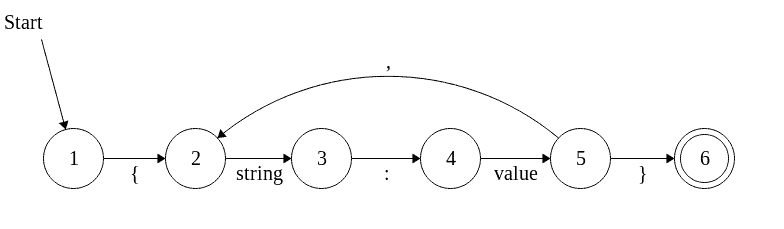## 1. Overview

If you’ve studied CS, you’ve undoubtedly taken a course about compilers or something similar; in these classes, the concept of Finite Automaton (also known as Finite State Machine) is taught. This is a way of formalizing the grammar rules of languages.

So how can this forgotten concept be helpful to us, high-level programmers, who don’t need to worry about building a new compiler?

Well, it turns out that the concept can simplify a lot of business scenarios and give us the tools to reason about complex logic.

As a quick example, we can also validate input without an external, third-party library.

## 2. The Algorithm

In a nutshell, such a machine declares states and ways of getting from one state to another. If you put a stream through it, you can validate its format with the following algorithm (pseudocode):

``````for (char c in input) {
if (automaton.accepts(c)) {
automaton.switchState(c);
input.pop(c);
} else {
break;
}
}
if (automaton.canStop() && input.isEmpty()) {
print("Valid");
} else {
print("Invalid");
}``````

We say the automaton “accepts” the given char if there is any arrow going from the current state, which has the char on it. Switching states means that a pointer is followed and the current state is replaced with the state that the arrow points to.

Finally, when the loop is over, we check if the automaton “can stop” (the current state is double-circled) and that input has been exhausted.

## 3. An Example

Let’s write a simple validator for a JSON object, to see the algorithm in action. Here is the automaton that accepts an object:Note that value can be one of the following: string, integer, boolean, null or another JSON object. For the sake of brevity, in our example, we’ll consider only strings.

### 3.1. The Code

Implementing a finite state machine is quite straightforward. We have the following:

``````public interface FiniteStateMachine {
FiniteStateMachine switchState(CharSequence c);
boolean canStop();
}

interface State {
State with(Transition tr);
State transit(CharSequence c);
boolean isFinal();
}

interface Transition {
boolean isPossible(CharSequence c);
State state();
}
``````

The relations between them are:

• The state machine has one current State and tells us if it can stop or not (if the state is final or not)
• A State has a list of Transitions which could be followed (outgoing arrows)
• A Transition tells us if the character is accepted and gives us the next State
``````publi class RtFiniteStateMachine implements FiniteStateMachine {

private State current;

public RtFiniteStateMachine(State initial) {
this.current = initial;
}

public FiniteStateMachine switchState(CharSequence c) {
return new RtFiniteStateMachine(this.current.transit(c));
}

public boolean canStop() {
return this.current.isFinal();
}
}``````

Note that the FiniteStateMachine implementation is immutable. This is mainly so a single instance of it can be used multiple times.

Following, we have the implementation RtState. The with(Transition) method returns the instance after the transition is added, for fluency. A State also tells us if it’s final (double-circled) or not.

``````public class RtState implements State {

private List<Transition> transitions;
private boolean isFinal;

public RtState() {
this(false);
}

public RtState(boolean isFinal) {
this.transitions = new ArrayList<>();
this.isFinal = isFinal;
}

public State transit(CharSequence c) {
return transitions
.stream()
.filter(t -> t.isPossible(c))
.map(Transition::state)
.findAny()
.orElseThrow(() -> new IllegalArgumentException("Input not accepted: " + c));
}

public boolean isFinal() {
return this.isFinal;
}

@Override
public State with(Transition tr) {
return this;
}
}``````

And finally, RtTransition which checks the transition rule and can give the next State:

``````public class RtTransition implements Transition {

private String rule;
private State next;
public State state() {
return this.next;
}

public boolean isPossible(CharSequence c) {
return this.rule.equalsIgnoreCase(String.valueOf(c));
}

// standard constructors
}``````

With this implementation, you should be able to build any state machine. The algorithm described at the beginning is as straightforward as:

``````String json = "{\"key\":\"value\"}";
FiniteStateMachine machine = this.buildJsonStateMachine();
for (int i = 0; i < json.length(); i++) {
machine = machine.switchState(String.valueOf(json.charAt(i)));
}

assertTrue(machine.canStop());``````

Check the test class RtFiniteStateMachineTest to see the buildJsonStateMachine() method. Note that it adds a few more states than the image above, to also catch the quotes that surround the Strings properly.

## 4. Conclusion

Finite automata are great tools that you can use in validating structured data.

However, they’re not widely known because they can get complicated when it comes to complex input (since a transition can be used for only one character). Nevertheless, they are great when it comes to checking a simple set of rules.

Finally, if you want to do some more complicated work using finite state machines, StatefulJ and squirrel are two libraries worth looking into.

You can check code samples on GitHub.

### Get started with Spring and Spring Boot, through the Learn Spring course:

>> CHECK OUT THE COURSE